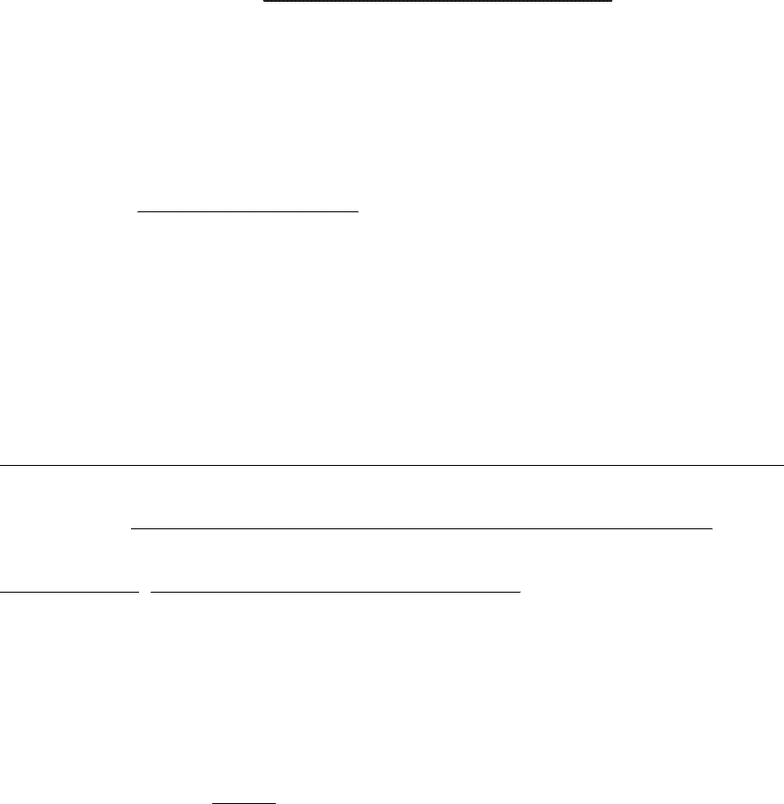Study Guides (390,000)
CA (150,000)
York (10,000)
ECON (900)
ECON 1010 (200)
All (70)
Midterm

# ECON 1010 Study Guide - Midterm Guide: Arc Elasticity, Indifference Curve, EcotaxExam

Department
Economics
Course Code
ECON 1010
Professor
All
Study Guide
Midterm

This preview shows pages 1-2. to view the full 7 pages of the document.- 1/7 -
PRINCIPLES OF MICROECONOMICS
Midterm Exam #2: Sample #1 Answers
Duration: 1 Hour
Non-programmable calculators only
Answer all questions on this test paper
INSTRUCTIONS:
This examination consists of TWO PARTS for a total of 100 marks
Part I 4 analytic/graphical questions for a total of 40%
Part II 5 multiple choice questions worth 2 marks each for a total of 10%. Wrong answers will
not be deducted from right in grading Part II.
All questions are to be answered in the spaces provided in this question paper booklet
Do not remove any pages or add any pages. No additional paper is supplied.
The blank backs of pages may be used for rough work. Show your work where applicable.
YOU MUST USE PEN INSTEAD OF PENCIL TO BE ELIGIBLE FOR REMARKING
Print your name and student number clearly on the front of the exam and on any loose pages.
Student Name: _______________________________________________________
(Family Name) (Given Name)
Student Number: ___________________________________
There are 7 pages to the exam.

Only pages 1-2 are available for preview. Some parts have been intentionally blurred.Principles of Microeconomics: Midterm Test #2, Sample #1 Answers
- 2/7 -
Part I
Clearly label all axes, curves, and points.
1. Incidence of a Tax: Equations (10 marks)
Suppose that the Demand and Supply equations for Radial Tires, a competitive market, are
P = 350 – 0.032Q and P = 90 + 0.048Q (Q is in tires and P is in \$s)
a) What is competitive equilibrium? (2 marks)
1 mark: Q = 3,250 from something like 350 – 0.032 = 90 + 0.048Q
1 mark: P = 246 from either P = 350 – 0.032*3250 or P = 90 + 0.048*3250
b) Suppose that the government now imposes a pollution tax of \$20 per tire to be collected by the
sellers. What is the new equilibrium price and quantity after the tax (in the short-run)? (3 marks)
1 mark: recognition that the new supply functions is P = 110 + 0.048Q
1 mark: Q = 3000 from something like 350 – 0.032 = 110 + 0.048Q
1 mark: P = 254 from either P = 350 – 0.032*3000 or P = 110 + 0.048*3000
c) What is the the Sellers’ Price after the tax is paid? (1 mark)
1 mark: Sellers’ Price = 234 from something like 254 – 20
d) What is the Sellers’ Share of the tax per tire? (1 mark)
1 mark: Sellers’ Share = 12 from something like 246 – 234 or 20 – 8
e) Calculate the Buyers’ Share of the tax without solving the equations. Show your work. (1 mark)
1 mark: Buyers Share = \$8 = \$20* 0.032/(0.032 + 0.048) [Must have something like this]
f) Calculate the tax revenue of the government from the \$20 tax per tire.
1 mark: Tax = 60,000 from something like 3000*20
g) What is the total expenditure by consumers on tires after the tax?
1 mark: = 762,000 from something like 254*3000